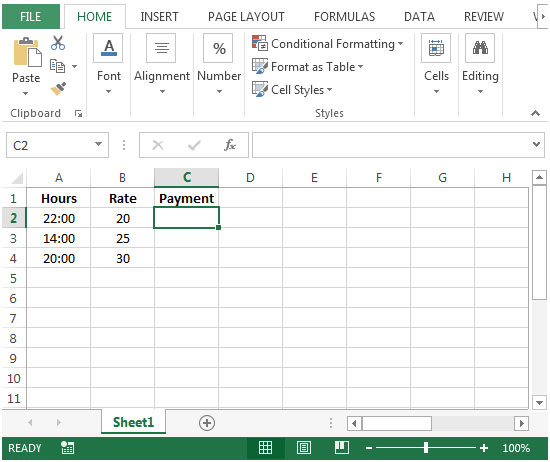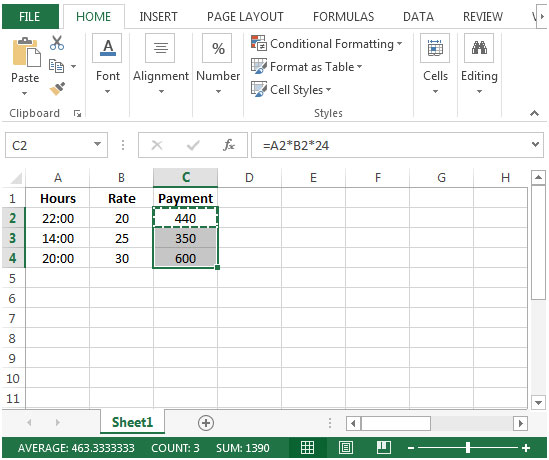# Multiplying Matrices in Microsoft Excel

To calculate the payment based on the worked hours & rate, we have to multiply each time value by its matching numerical rate.

Let us take an example:

In this example, we will show you how to calculate the number of working hours say 22 hours, & do a simple multiplication with hourly rate to get the payment.

• Column A contains time values representing worked hours.
Column B contains hourly rates.• In order to calculate the payment, we will multiply the hours worked with rate.
• In cell C2, the formula would be =A2*B2*24
• The function will return 0:00, so we have to change the cell formatting.
• Press Ctrl+1, format cells dialog box will appear.
• Click on Numbers tab > General>Click on ok.• Copying down the formula from cell C2 to range C3:C4, we will get the desired result.In this tip, Hours x Rate will be multiplied by 24 because Excel treats time formats quite differently than regular numbers.

One day = one whole time unit, therefore one hour = 1/24th of a whole unit, and we must multiply hours by 24 to achieve whole units of time.We would love to hear from you, do let us know how we can improve, complement or innovate our work and make it better for you. Write us at info@exceltip.com

## Users are saying about us...

1.This is excellent, it works even for minutes and seconds.. Thanks man...

2.Thank you for the help. This really works.

3.ebtisam ebrahim

Thanks a lot
its work for me and my problem solved

4.thanks a lot

Terms and Conditions of use

The applications/code on this site are distributed as is and without warranties or liability. In no event shall the owner of the copyrights, or the authors of the applications/code be liable for any loss of profit, any problems or any damage resulting from the use or evaluation of the applications/code.